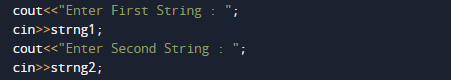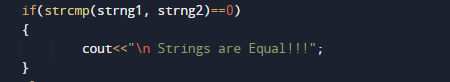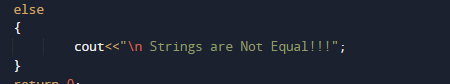C++ Program to Compare Two Strings

In this tutorial you will learn about the C++ Program to Compare Two Strings and its application with practical example.

In this tutorial, we will learn to create a C++ program that will Compare two Strings using C++ programming.

Prerequisites

Before starting with this tutorial we assume that you are best aware of the following C++ programming topics:

• Operators.
• looping statements.
• Basic input/output.
• Basic c++ programming
• For Loop.
• String.

String

Usually”string,” means a collection of characters called ‘string’ that represents text data in languages like c,c++ and Java.

What is array?

An Array is a collection variable elements  that can store multiple values in a single variable of same type.

C++ Program to Compare two Strings.

In this program we will compare two strings. We would first declared and initialized the required variables. Next, we would prompt user to input two strings .Later we will compare  strings.

outputIn the above program, we have first declared and initialized a set variables required in the program.

• strng1 = it will hold entered string one.
• strng2 = it will hold entered string two.

In our program, we will compare two string if both are similar we will print string are equal if not they are not equal.

First of all we  take two strings input from user and store their value in variable strng1 and strng2.And now with the help of strcmp() function we will compare these two strings .we check the string in if else condition if string are equal strcmp() gives true valueand if string are not equal strcmp() gives false value.Here Strcmp()function is a pre-defined function in a string.h header file.The strcmp() function take two strings as a arguments, and returns an integer value where the integer value can be zeropositive or Negative.

On comparing the strings by using the strcmp() function, i.e., strcmp(str1,str2). This function will compare both the strings strng1 and strng2. the function returns 0 if values of strings are equal ,means that both the strings are same, otherwise the strings are not equal.

In this tutorial we have learn about the C++ Program to Compare Two Strings and its application with practical example. I hope you will like this tutorial.